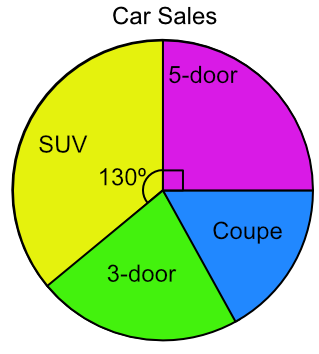Pie Charts

# Pie Charts

GCSE(F),

A Pie Chart shows data as sectors of a circle. A pie chart is built from a table of data.

Building a pie chart consists of these steps:

• Add the frequencies to obtain a total;

• Divide 360º by the total (to get the number of degrees for one item);

• Multiply each frequency by this number;

• Plot the pie chart using these values;

• Label the pie chart and provide a title

## Examples

1. A school is taking 120 students on a trip. The students come from several year groups. Plot a pie chart to represent the data.

Frequency
Year 721
Year 827
Year 929
Year 1032
Year 1111

Answer:Add frequencies for total = 120.

Each student is worth 360 ÷ 120 = 3º

Multiply each frequency by 3º

Frequency
Year 7213 x 21 = 63
Year 8273 x 27 = 81
Year 9293 x 29 = 87
Year 10323 x 32 = 96
Year 11113 x 11 = 33
Year Total120360

Plot the chart, adding a title and labels

2. The pie chart below shows the type of cars sold by a car showroom over one month. 18 5-door cars were sold. How many SUVs were sold?